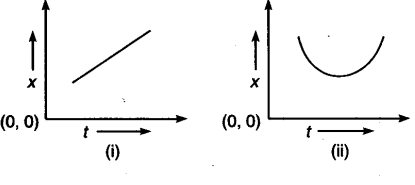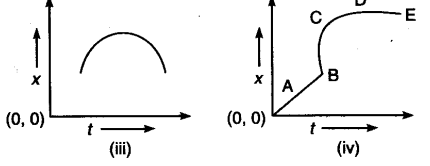# Identify the case/cases of positive, negative or zero acceleration in the following curves :

Identify the case/cases of positive, negative or zero acceleration in the following curves :(i) Since, position is increasing with time so it is a case of positive acceleration.
(ii) The upward curve of the given graph indicates positive acceleration.
(iii) The drooping of curve i.e. downward curve indicates negative acceleration.
(iv) OABCD represents positive acceleration, DE
represents zero acceleration.